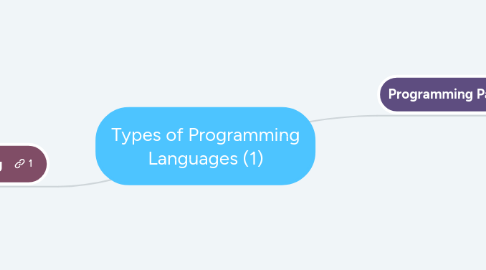# Types of Programming Languages (1)Daniel Stone
Get Started. It's FreeTypes of Programming Languages (1)### 1.1. Imperative Paradigms: First do this and next do that.

1.1.1. The 'first do this, next do that' is a short phrase which really in a nutshell describes the spirit of the imperative paradigm. The basic idea is the command, which has a measurable effect on the program state. The phrase also reflects that the order to the commands is important. 'First do that, then do this' would be different from 'first do this, then do that'.

1.1.1.1. Characteristics: - Discipline and data - Incremental change of the state as a function of time. - Execution of steps in an order governed by control structures. (command steps) - Straightforward abstraction (Von Neumann computer works) - Language representatives : Fortran, Algol, Pacal, Basic, C - The natural abstraction is the procedure - Abstract one or more actions to a procedure (Single command), "Procedural Programming)

### 1.2. Functional Paradigms: Evaluate an expression and use the resulting value for something.

1.2.1. Functional programming is in many respects a simpler and more clean programming paradigm than the imperative one. The reason is that the paradigm originates from a purely mathematical discipline: the theory of functions.

1.2.1.1. Characteristics: - Discipline and idea - Mathematics and the theory of functions - The values produced are non-mutable - Impossible to change any constituent of a composite value - As a remedy, it is possible to make a revised copy of composite value - Atemporal - Time only plays a minor role compared to the imperative paradigm - Applicative - All computations are done by applying (calling) functions - The natural abstraction is the function -Abstracts a single expression to a function which can be evaluated as an expression - Functions are first class values - Functions are full-fledged data just like numbers, lists, ... - Fits well with computations driven by needs - Opens a new world of possibilities

### 1.3. Logic Paradigms: Answer a question via search for a solution

1.3.1. The logic paradigm is dramatically different from the other three main programming paradigms. The logic paradigm fits extremely well when applied in problem domains that deal with the extraction of knowledge from basic facts and relations. The logical paradigm seems less natural in the more general areas of computation.

1.3.1.1. Characteristics: - Discipline and idea - Automatic proofs within artificial intelligence - Based on axioms, inference rules, and queries. - Program execution becomes a systematic search in a set of facts, making use of a set of inference rules

### 1.4. Object-oriented Paradigm: Send messages between objects to simulate the temporal evolution of a set of real world phenomena.

1.4.1. The primary for object-oriented paradigms strong support of encapsulation and the logical grouping of program aspects. An object-oriented program is constructed with the outset in concepts, which are important in the problem domain of interest. In that way, all the necessary technicalities of programming come in second row.

1.4.1.1. Characteristics: - Discipline and idea - The theory of concepts, and models of human interaction with real world phenomena - Data as well as operations are encapsulated in objects - Information hiding is used to protect internal properties of an object - Objects interact by means of message passing - A metaphor for applying an operation on an object - In most object-oriented languages objects are grouped in classes - Objects in classes are similar enough to allow programming of the classes, as opposed to programming of the individual objects - Classes represent concepts whereas objects represent phenomena - Classes are organized in inheritance hierarchies - Provides for class extension or specialization

## 2. Procedural Programming

### 2.1. - Problem orientated - Describe the problem and how to solve it - Solution divided into procedures

2.1.1. Procedural Programming: A program where instructions are given in sequence; selection is used to decide what a program does and iteration dictates how many times it does it. In procedural programming, programs are broken down into key blocks called procedures and functions. Examples of procedural languages include Basic, C and Pascal

2.1.2. Logic Programming: Rather than stating what the program should do, in logic programming a problem is expressed as a set of facts (Things that are always true) and rules (Things that are true if particular facts are true.) These facts and rules are then used to find a given goal. The most commonly used logic language is 'Prolog'.

2.1.3. Functional Programming: A function, in mathematics, takes in a value or values and returns a value, for example: double(4) would return 8 Highestcommonfactor(36,24) would return 12 In functional programming, a description of the solution to a problem is built up through a collection of functions. Example include Haskell and ML.